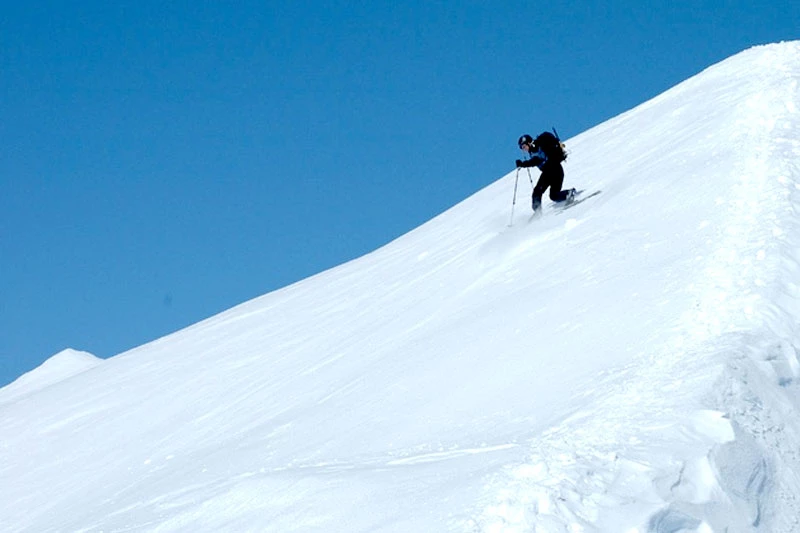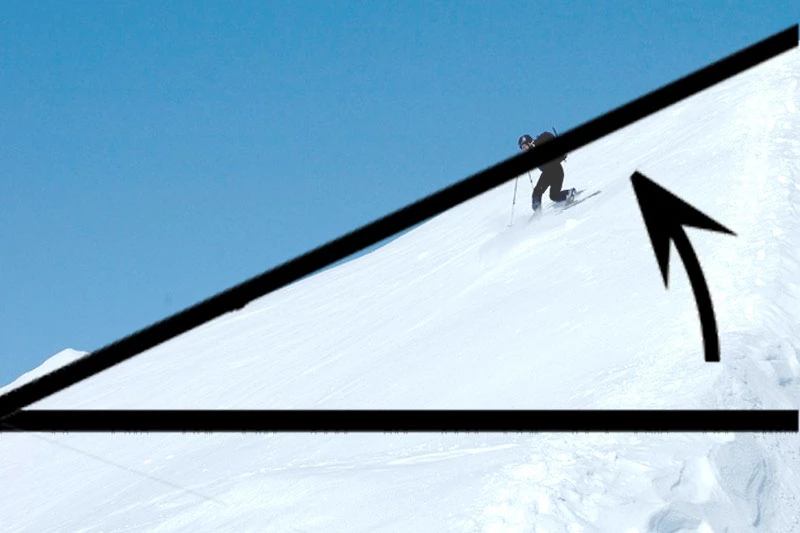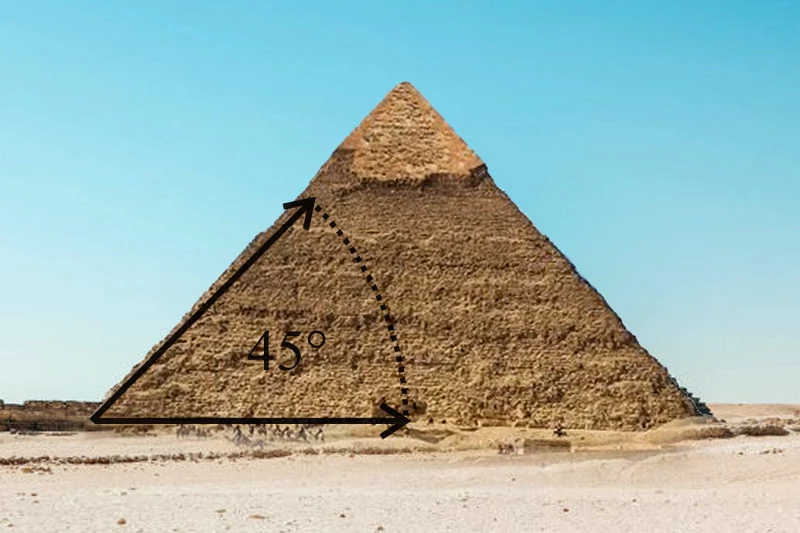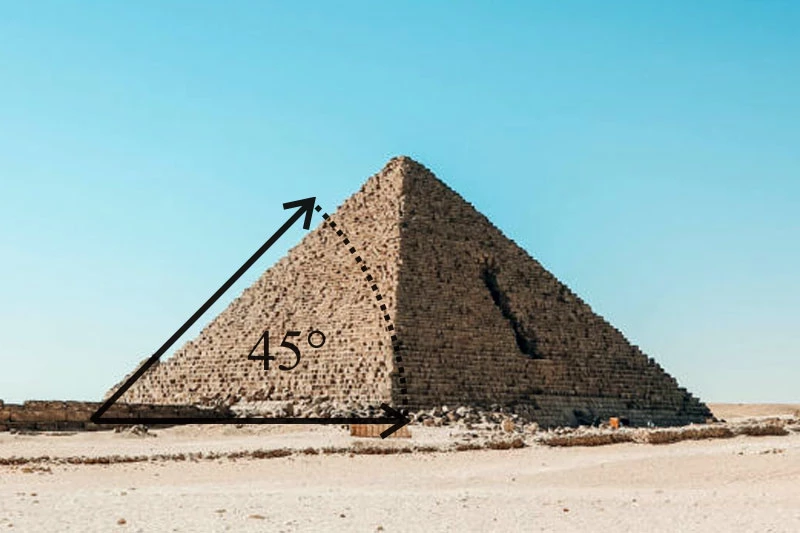# Giza Pyramid Slope Angle

The Giza Pyramid Slope Angle, expressed in degrees, is an angle of inclination, measuring steepness. Below are images: mountain slope (left), and angle made clear (right).Drawing straight lines, we can determine the angle of slope by measuring rise and run. Rise is the difference vertically, while run is the distance horizontally. Drawing a line at a diagonal allows us to determine the slope.

top

#### Giza Pyramid Slope Angle (The Slope)

Every pyramid has two slopes; face slope and edge slope.When considering pyramid slope, we must distinguish between edge and face. Using the two pyramid images above as examples, there are two perspectives from which to examine the slope of a pyramid; face (left) and edge (right).

top

#### Giza Pyramid Slope Angle (The Face)

The first perspective (above, left) is with a pyramid face directly in front of us. Viewed from this perspective, the Giza Pyramid Slope Angle (Face) is 51.83 degrees (51 degrees, 50 minutes, etc.). When we speak about the special slope of the Giza pyramid, we are referring to this 51.83 degrees. This slope is related to the Golden Ratio (or Phi).

#### Giza Pyramid Slope Angle (The Edge)

The second perspective is with a corner facing us. This will allow us to observe the edge slope. Viewed from this perspective, the Giza Pyramid Slope Angle (Edge) is 42 degrees (41 degrees, 59 minutes, etc.).

Related Subjects:

topIFP Customers (U.S.)
Large
Medium

Large
Medium
Small

## Subscribe to IFP emails

(for discounts, specials, etc.)

* indicates required

### Inner Fire Pyramids